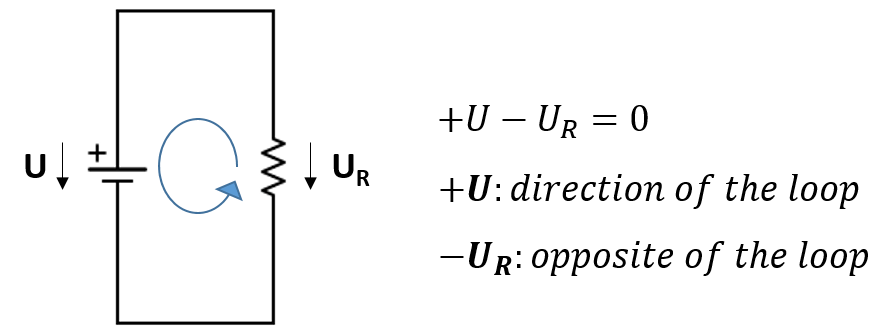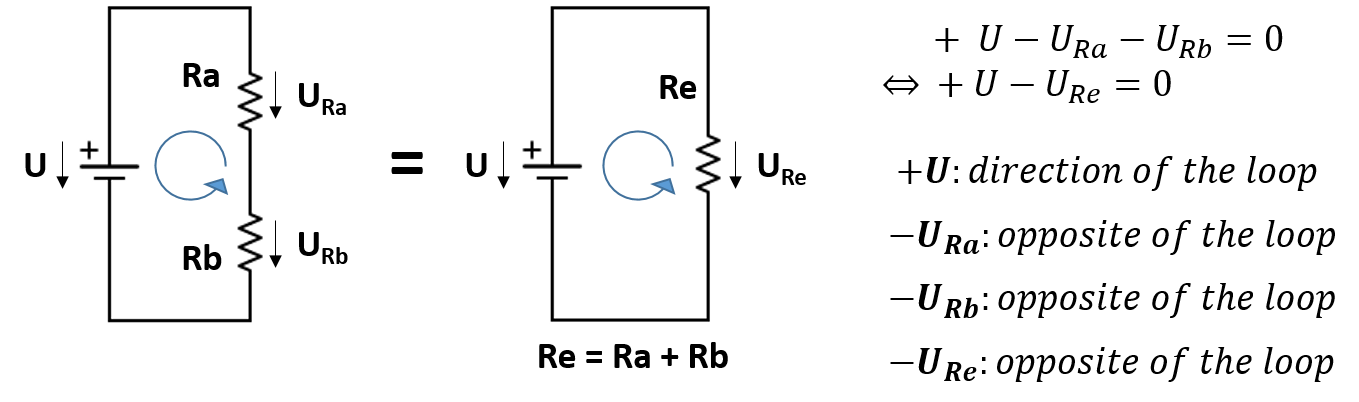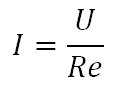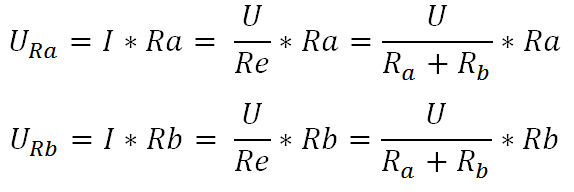# Laws of electronics: KVL

This post describes one of the basic laws of circuit analysis, more precisely KVL (Kirchhoff’s Voltage Law), which states that the sum of all voltages in a closed loop of a circuit are equal to zero.

KVL

Kirchhoff’s Voltage Law states that the sum of all voltages in a closed loop of a circuit (taking into account the magnitude and the signal) are equal to zero.

So, as mentioned, we need to take into consideration the signal of the voltage, which needs to be defined according to some principle. To do so, we define a “direction” for the loop and consider that every voltage drop on that direction is positive and in the opposite direction is negative.

The law yields true if we choose the clockwise or the counterclockwise direction. As long as we maintain the same direction for the loop to define the signal of the voltage drops, the sum will be zero. Figure 1 shows a simple example for a resistive circuit.Figure 1 – Simple circuit with demonstration of KCL.

If we analyse a circuit with 2 resistors in series, as shown in figure 2, the law will still work. First, we take into consideration that 2 resistors in series can be simplified to 1 resistor whose value is the sum of both.Figure 2 – Resistors in series with application of KVL.

From that simplification and using Ohm’s law, we get the current flowing in the circuit from the voltage applied to the equivalent resistor:Knowing the total current and using again Ohm’s law in the circuit before the simplification, we get the voltage drop in each individual resistor:Finally, we consider a loop in the counterclockwise direction as shown in figure 3 and we can confirm that KVL is verified:Although we used simple circuits to demonstrate the law, this can be applied to more complex ones.

One important thing thing is that KVL is derived from Maxwell’s equations, which are the fundamental equations for electricity and magnetism. Nevertheless, the mathematical operations needed to derive KVL from them and are not trivial and are outside the scope of this post.